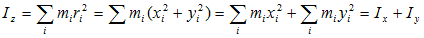# Perpendicular Axis Theorem

## Perpendicular axis theorem

• This theorem is applicable only to the plane laminar bodies
• This theorem states that, the moment of inertia of a plane laminar about an axis perpendicular to its plane is equal to the sum of the moment of inertia of the lamina about two axis mutually perpendicular to each other in its plane and intersecting each other at the point where perpendicular axis passes through it
• Consider plane laminar body of arbitrary shape lying in the x-y plane as shown below in the figure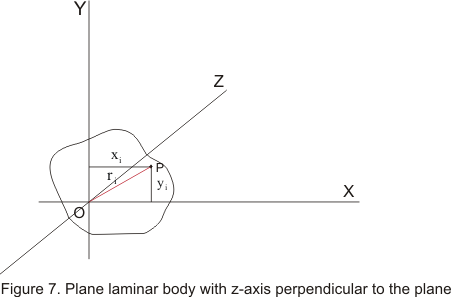• The moment of inertia about the z-axis equals to the sum of the moments of inertia about the x-axis and y axis
• To prove it consider the moment of inertia about x-axis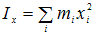where sum is taken over all the element of the mass mi
• The moment of inertia about the y axis is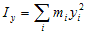• Moment of inertia about z axis is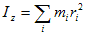where ri is perpendicular distance of particle at point P from the OZ axis
• For each element
ri2=xi2 + yi2